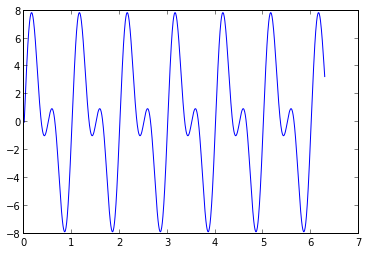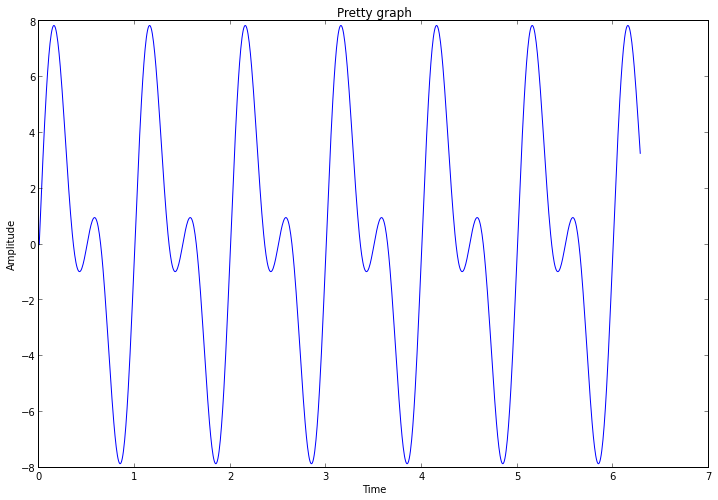# Big graphs in IPython Notebook

I've been doing a good bit of graphing in IPython Notebook recently, and I often wanted to make the graphs larger. I also often wanted to label the graph axes. So I wrote this simple function and have been using it a lot.

# Graphing helper function
def setup_graph(title='', x_label='', y_label='', fig_size=None):
fig = plt.figure()
if fig_size != None:
fig.set_size_inches(fig_size, fig_size)
ax.set_title(title)
ax.set_xlabel(x_label)
ax.set_ylabel(y_label)


Here's a demo of using it...

In :
x = linspace(0, 2 * pi, 1000)
y = 5 * sin(1 * 2*pi*x) + 4 * sin(2 * 2*pi*x)


## Without setup_graph()

In :
_ = plot(x, y)## With setup_graph()

In :
setup_graph(title='Pretty graph', x_label='Time', y_label='Amplitude', fig_size=(12,8))
_ = plot(x, y)